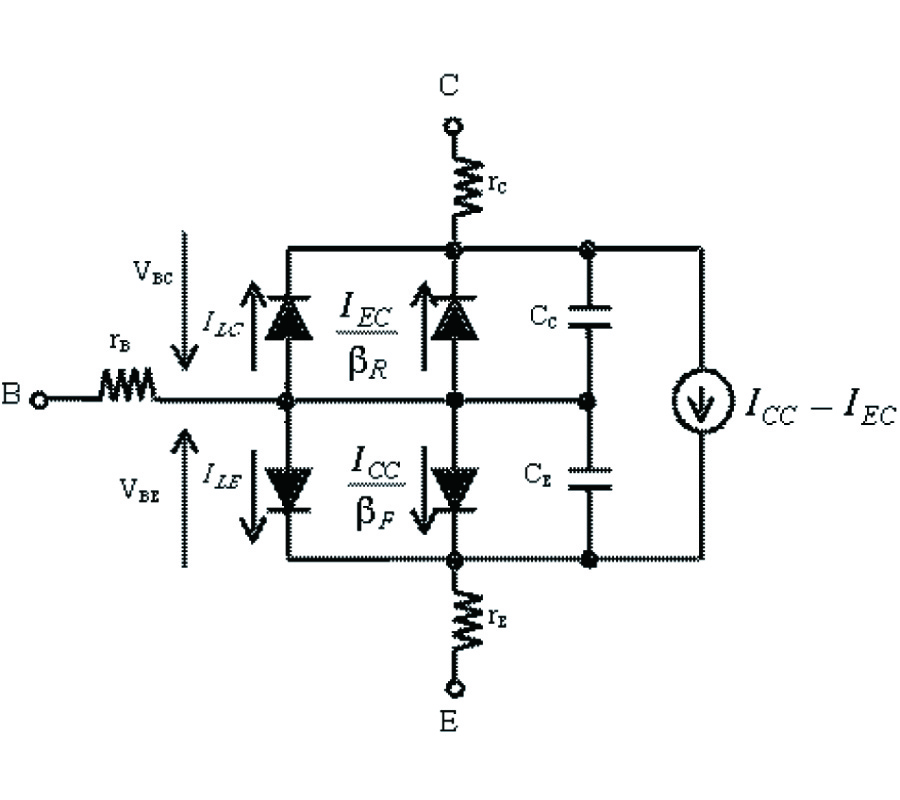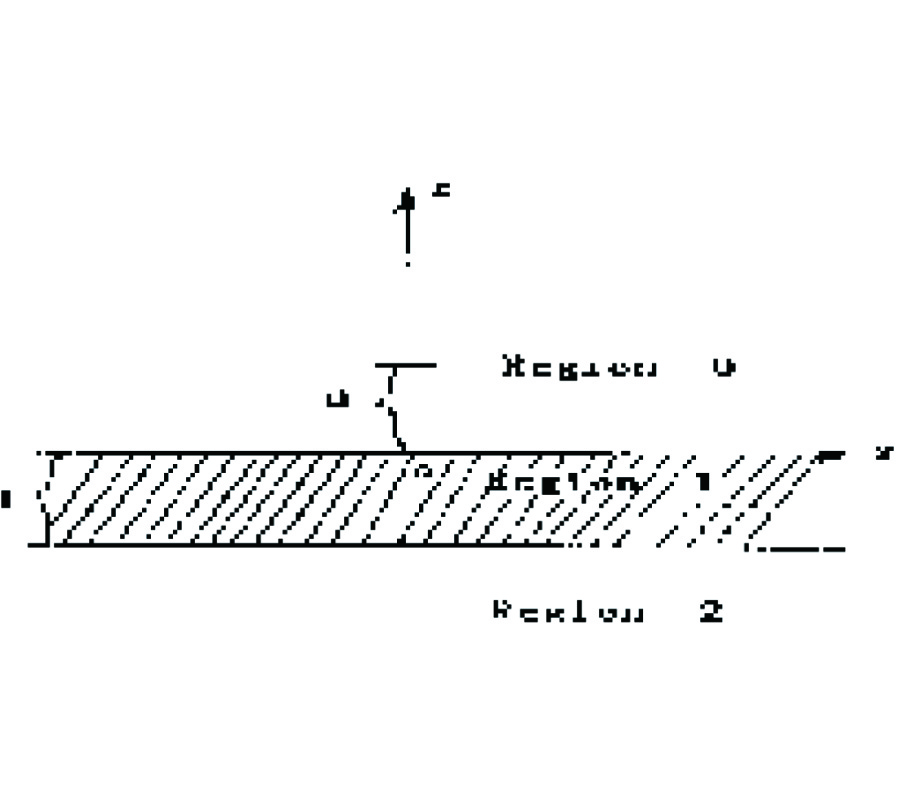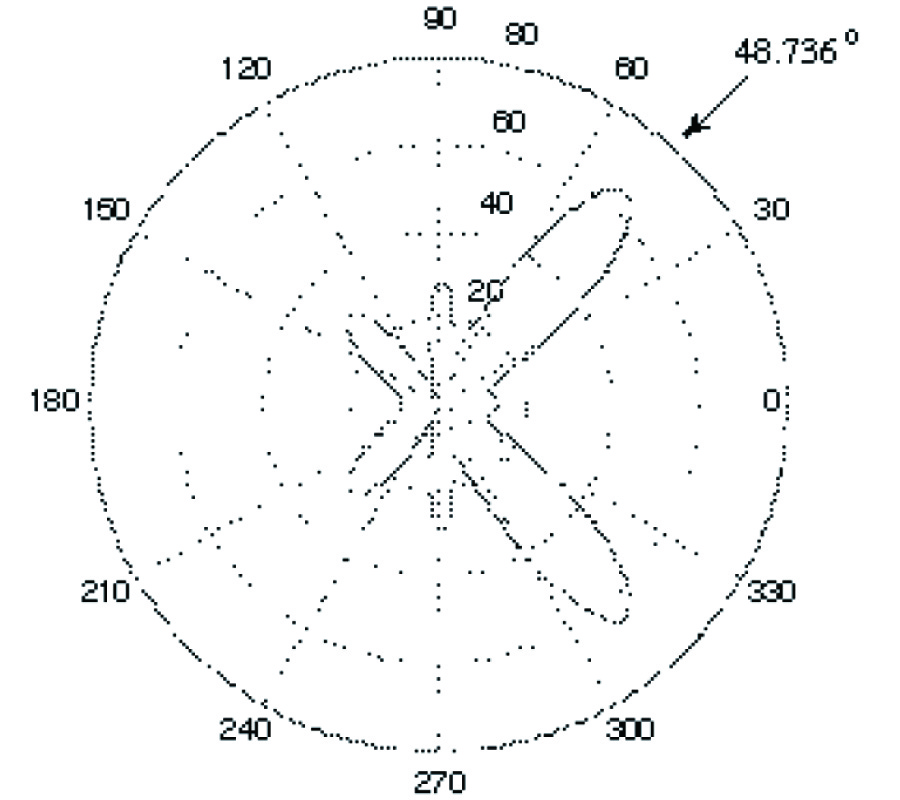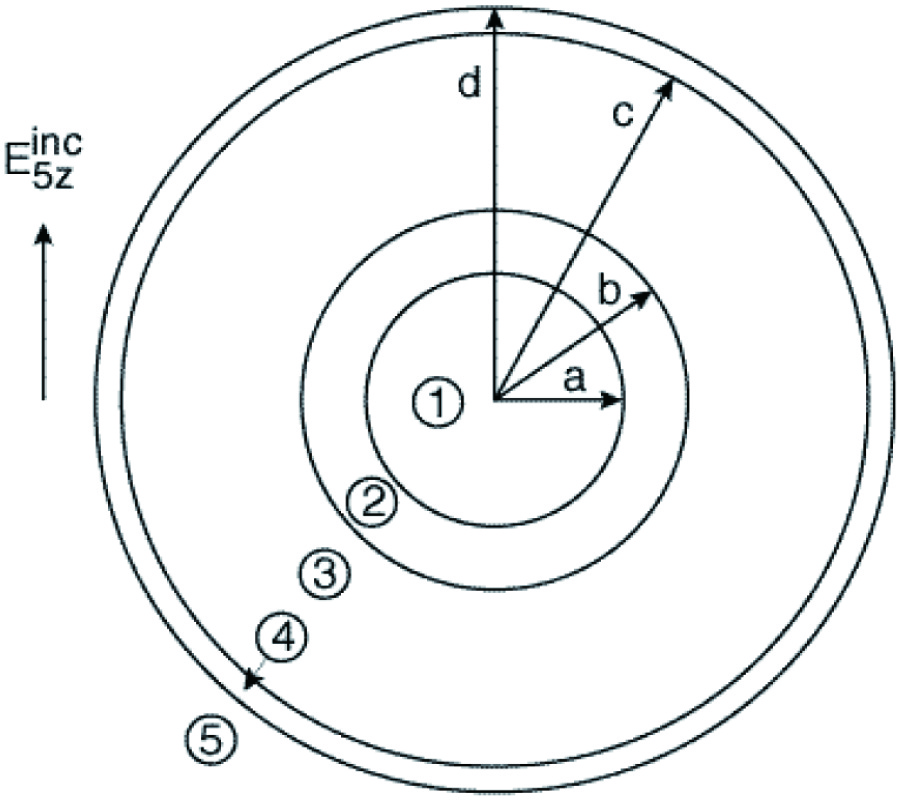ISSN: 1070-4698

# Vol. 36

Latest Volume
All Volumes
All Issues

PIER Vol. 36, 319-335, 2002. doi:10.2528/PIER02062101

### Mode-Matching Analysis of Waveguide T-Junction Loaded with an h -Plane Dielectric Slab

Z. Jiang, Z. Shen, and X. Shan

This paper presents a full-wave mode-matching analysis of a rectangular waveguide T-junction partially loaded with an H-plane dielectric slab. First, the longitudinal section electric (LSE) and longitudinal section magnetic (LSM) modes in an H-plane dielectric-filled rectangular waveguide are obtained. Second, the dielectric-loaded Tjunction is then divided into four regions and their electromagnetic fields are expanded into the summation of their modal functions using the resonator method. Finally, a mode-matching process for the tangential field components across regional interfaces is carried out to derive the generalized scattering matrix of the waveguide T-junction. Using the cascading connection technique of generalized scattering matrix, various waveguide couplers and power dividers/combiners with H-plane dielectric slabs can be easily analyzed and designed. Numerical results are presented and compared with those obtained by measurement or Ansoft's HFSS. Good agreement is observed.

PIER Vol. 36, 279-317, 2002. doi:10.2528/PIER02032604

###A Variable Metric Electrodynamics. Plane Waves

Bernard Jancewicz

Classical electrodynamics can be divided into two parts. In the first one, a need of introducing a plenty of directed quantities occurs, namely multivectors and differential forms but no scalar product is necessary. We call it premetric electrodynamics. In this part, principal equations of the theory can be tackled. The second part concerns solutions of the equations and requires establishing of a scalar product and, consequently, a metric. For anisotropic media two scalar products can be introduced depending on the electric permittivity and magnetic permeability tensors. In the case of plane electromagnetic waves both of them are needed because two constitutive equations are needed: one for the electric fields, the other for the magnetic field. We show which part of the description of plane electromagnetic waves is independent of scalar products, and where they become necessary.

PIER Vol. 36, 265-278, 2002. doi:10.2528/PIER02022102

###Design of Dual-Frequency Microstrip Patch Antennas and Application for IMT-2000 Mobile Handsets

Y. J. Wang and C. Lee

Two major objectives are concerned in this paper. On one hand, a multiplicity of typical methodologies available for achieving dual-frequency operation for microstrip patch antennas are summarized and classified into three categories, based on their intrinsic mechanisms. On the other hand, by employing the dual-frequency solutions, microstrip patch antennas are applied to the mobile handsets of the third-generation IMT-2000 system. Two novel microstrip patch antennas with broadband property and miniaturized size have been proposed and discussed theoretically and experimentally. Only a single simply-slotted patch, either semi-disc or square, is introduced in each of the two probe-fed antennas. Both antennas exhibit the impedance bandwidths (return loss · −10 dB) of 25.6% and 34.4% respectively, which would be suitable for the practical application of the IMT-2000 mobile handsets.

PIER Vol. 36, 247-264, 2002. doi:10.2528/PIER02021701

###Electromagnetic Coupling Analysis of Transient Signal through Slots OR Apertures Perforated in a Shielding Metallic Enclosure Using FDTD Methodology

Ya Wang, Wee Jin Koh, C. Lee, and Kye See

The paper presents electromagnetic coupling of an electrical fast transient plane wave penetrating through slots or apertures perforated in one side of a shielding metallic enclosure. Numerous slots and apertures of different configurations and dimensions have been developed, which include a single slot of different length, a single aperture of different width, multiple-angular apertures of different geometry, multiple-cell aperture of different cell numbers, multiple thin slots, an aperture-cell array making up the whole side of the enclosure, and a miscellany case simulating a PC main frame. FDTD numerical method is applied to the EMI/EMC model, while time-domain outputs are converted to frequency-domain responses for further analyses using a FFT program. Practical conclusions and recommendations are drawn to aid shielding enclosure design and electromagnetic interference protection.

PIER Vol. 36, 193-246, 2002. doi:10.2528/PIER02021702

###A Survey of Various Frequency Domain Integral Equations for the Analysis of Scattering from Three-Dimensional Dielectric Objects

Baek-Ho Jung, Tapan Kumar Sarkar, and Y.-S. Chung

In this paper, we present four different formulations for the analysis of electromagnetic scattering from arbitrarily shaped three-dimensional (3-D) homogeneous dielectric body in the frequency domain. The four integral equations treated here are the electric field integral equation (EFIE), the magnetic field integral equation (MFIE), the combined field integral equation (CFIE), and the PMCHW (Poggio, Miller, Chang, Harrington, and Wu) formulation. For the CFIE case, we propose eight separate formulations with different combinations of expansion and testing functions that result in sixteen different formulations of CFIE. One of the objectives of this paper is to illustrate that not all CFIE are valid methodologies in removing defects, which occur at a frequency corresponding to an internal resonance of the structure. Numerical results involving the equivalent electric and magnetic currents, far scattered fields, and radar cross section (RCS) are presented for three canonical dielectric scatterers, viz. a sphere, a cube, and a finite circular cylinder, to illustrate which formulation works and which does not.

PIER Vol. 36, 179-192, 2002. doi:10.2528/PIER02013001

###Modeling of Bipolar Junction Transistor in FDTD Simulation of Printed Circuit Board

F. Kung and H. T. Chuah

A simple and efficient approximate method to incorporate nonlinear bipolar junction transistor (BJT) into Finite-Difference Time-Domain (FDTD) framework is presented. This method applies Taylor expansion on the nonlinear transport equations of the BJT based on Gummel-Poon model . The results are two coupled one-step explicit finite difference schemes for the electromagnetic fields in the vicinity of the BJT, which can be solved easily. A simulation example is carried out for a power amplifier and the result compares well with the measurement. A two-step simulation scheme is introduced to hasten the process of reaching transient steady state. Finally, brief comments on treating the FDTD framework as a dynamical system is included. This perspective is useful for analyzing the stability of FDTD framework with nonlinear lumped elements.

PIER Vol. 36, 153-177, 2002. doi:10.2528/PIER02012503

###Validation and Numerical Convergence of the Hankel-Bessel and Mathieu Rigorous Coupled Wave Analysis Algorithms for Radially and Azimuthally --- Inhomogeneous, Elliptical, Cylindrical Systems

John Jarem

A Rigorous Coupled Wave Analysis (RCWA) algorithm for electromagnetic (EM) scattering from radially and azimuthally inhomogeneous material elliptical systems based on State Variable (SV) techniques and based on circular-cylindrical Hankel-Bessel expansion modes is developed for the first time. The algorithm in conjunction with the elliptical system RCWA algorithm , which was based on SV techniques and Mathieu expansion modes, is used to validate and study numerical convergence of both elliptical RCWA algorithms. The formulation of the SV, Hankel-Bessel elliptical algorithm is presented. Two numerical elliptical examples are studied in detail by both algorithms, a homogeneous one which consists of three different uniform materials located in three elliptical regions and an inhomogeneous one which consists of an azimuthal, dielectric, step profile which is located between two uniform material elliptical regions. In this paper EM field scattering from a step profile which possessed a much larger dielectric step profile difference than was studied in  is presented. Validation and numerical convergence data of the Hankel-Bessel and the Mathieu  RCWA algorithm is presented for the first time, both in plot figures and in tables, when different numbers of expansion modes were used, when different number of layers were used, and when different numbers of SV harmonics were used. Validation of the RCWA algorithms was further carried out for the homogeneous case, by using Mathieu expansion modes in all regions and was carried out by using Hankel-Bessel expansion modes and Mathieu expansion modes in different regions. Validation of the Hankel-Bessel and Mathieu  RCWA algorithms was observed to a high degree of accuracy. It was found for the numerical example tested, that the number of modes used in the RCWA algorithms needed to exceed a critical minimum value in order to obtain meaningful, accurate results, and after this critical number of modes was exceeded, that convergence occurred rapidly as the number of modes increased. It was also found that as the number of layers used in the algorithm increased that the numerical accuracy of the RCWA solution slowly increased.

PIER Vol. 36, 139-152, 2002. doi:10.2528/PIER02011801

###The Electromagnetic Field Produced by a Horizontal Electric Dipole Over a Dielectric Coated Perfect Conductor

J. L. Tang and W. Hong

The analytical expressions for the electromagnetic field generated by a horizontal electric dipole over a dielectric coated perfect conductor are derived by transformation of integral path. From the expressions, it can be clearly observed that the excited field consists of the direct wave, reflected wave, trapped surface wave and lateral wave. The propagation wave number of trapped surface wave, which depends on electric parameters and thickness of the dielectric layer, is between the wave number k0 and k1.

PIER Vol. 36, 121-137, 2002. doi:10.2528/PIER02011001

### Volterra Differential Constitutive Operators and Locality Considerations in Electromagnetic Theory

Dan Censor and Timor Melamed

Macroscopic Maxwell's theory for electrodynamics is an indeterminate set of coupled, vector, partial differential equations. This infrastructure requires the supplement of constitutive equations. Recently a general framework has been suggested, taking into account dispersion, inhomogeneity and nonlinearity, in which the constitutive equations are posited as differential equations involving the differential operators based on the Volterra functional series. The validity of such representations needs to be examined. Here it is shown that for such representations to be effective, the spatiotemporal functions associated with the Volterra differential operators must be highly localized, or equivalently, widely extended in the transform space. This is achieved by exploiting Delta-function expansions, leading in a natural way to polynomial differential operators. The Four-vector Minkowski space is used throughout, facilitating general results and compact notation.

PIER Vol. 36, 101-119, 2002. doi:10.2528/PIER01100302

###Electronic Beam Steering Using Switched Parasitic Smart Antenna Arrays

Pantelis Varlamos and Christos N. Capsalis

A method of designing smart antennas based on switched parasitic antenna arrays is presented in this paper. The direction of maximum gain can be controlled by a digital word, while the selection of element spacing and weighting is optimized using the method of genetic algorithms. Various results are presented to show how antennas of this type perform, outlining the advantages and limitations of their design.

PIER Vol. 36, 81-100, 2002. doi:10.2528/PIER01060602

###Self-Field ac Power Dissipation in High-Tc Superconducting Tapes and a Prototype Cable

S. A. Awan and S. Sali

The measured self-field AC power dissipation in superconducting BiSrCaCuO-2223/Ag tapes and a prototype cable is cable is compared with theoretical models. A brief overview of the theoretical background for AC loss calculations in superconductor tapes with different geometrical shapes is also discussed. New models for the harmonic components of the fundamental frequency and the current dependent non-linear inductance are also derived. It is shown that the latter models can be used to estimate current distribution and the variation of flux penetration in superconducting tapes. Two separate experimental apparatus were designed and constructed for measurements on tapes and prototype cable systems. The observed losses in tapes are reasonably well described by models based on the Critical State Model (CSM). In contrast, the measured losses in the prototype cable are found to be a factor of approximately two higher the predicted values. Further investigations showed that this may be due to inhomogeneous contact resistance between individual tapes and the current joints and the variation in critical current density (JC) distribution between tapes. The significance of current dependence of the loss component, inductive quadrature component, phase error in measurements and the definition of the critical current in the prototype cable are also discussed.

PIER Vol. 36, 61-79, 2002. doi:10.2528/PIER02031501

###The Low-Frequency Electric Fields Induced in a Spherical Cell Including Its Nucleus

Ronold W. P. King and Dionisios Margetis

After a review of work leading to the determination of the electric field induced in the human body when exposed to the electromagnetic field near an extremely-low-frequency high-voltage transmission line, attention is directed to a spherical cell exposed to the electric field in the body. Following a brief discussion of the potential biological significance of the study, the electric field acting on the surface of the cell and the electric field induced by it in the region between the outer cell membrane and the nuclear envelope are determined analytically, as is the field induced in the nucleus. It is shown, as an example, that when the body is exposed to a 60-Hz axial electric field of 2100 V/m, the field induced in the nucleus of a cell in the body is 0.27 nV/m when the radius of the nucleus is half that of the cell. The biologically interesting electric field along the outer surface of the nuclear envelope is in this case 2.7 μV/m. The simple analytical formulas can be applied to other values of the parameters, such as varying sizes of the nucleus (see Figure 2), and to power-line fields of different magnitude.

PIER Vol. 36, 21-59, 2002. doi:10.2528/PIER01110502

### Theory of Optical Bullets

This paper is a theoretical study of solitons in multidimensions, also known as optical bullets, that is governed by the nonlinear Schrodinger's equation in 1 + 3 dimensions. The parameter dynamics of such multidimensional solitons has been obtained. The study is extended to obtain the adiabatic evolution of soliton parameters in presence of the perturbation terms. Furthermore, the parameter dynamics for the vector multidimensional solitons and including the presence of the perturbation terms has been obtained.

PIER Vol. 36, 1-19, 2002. doi:10.2528/PIER01110203

###Optimal Synthesis of Line Source Antennas Based on Rhodes Distributions

Julio C. Bregains, J. A. Rodriguez, and F. Ares

In this paper we show that by means of an appropriate optimization technique the zeros of Rhodes radiation patterns can be perturbed so as to improve or modify pattern and/or the aperture distribution characteristics without altering the behaviour of the excitation amplitude distribution at the ends of the aperture. The examples presented include symmetric and asymmetric shaped beams, sum patterns with individually controlled side lobe heights, shaped beams generated by real excitations, and sum pattern aperture distributions that are smoother than the original Rhodes distribution.